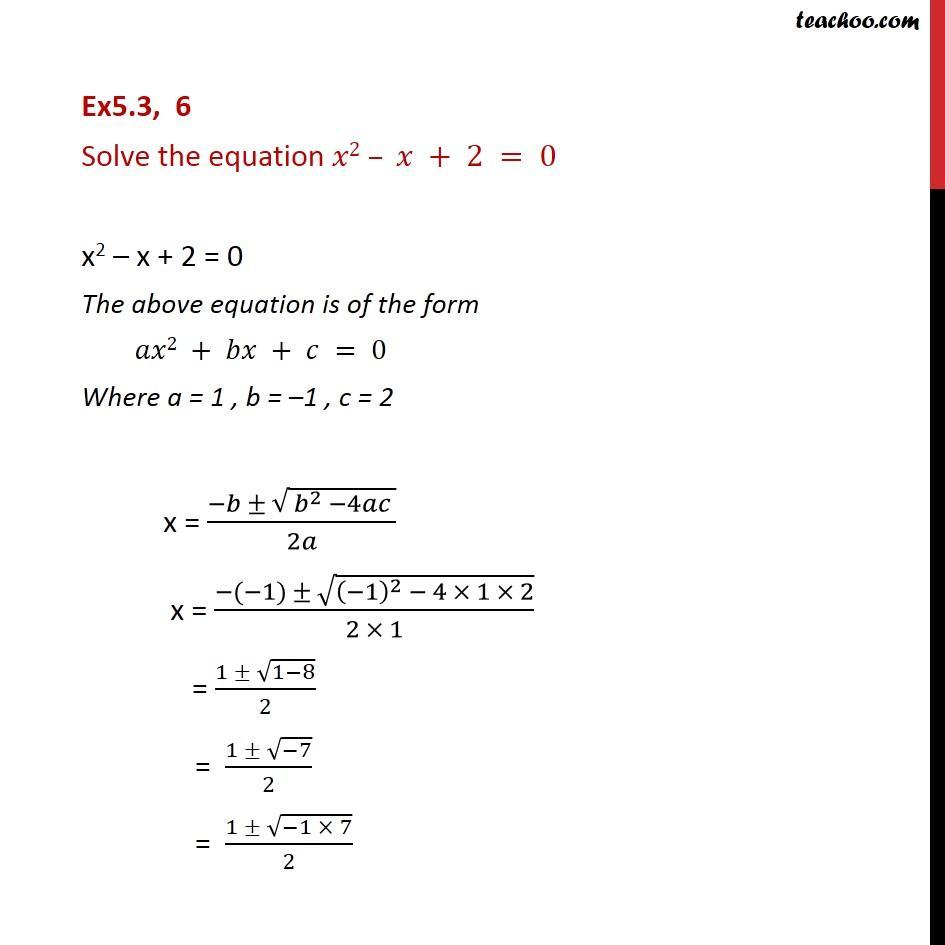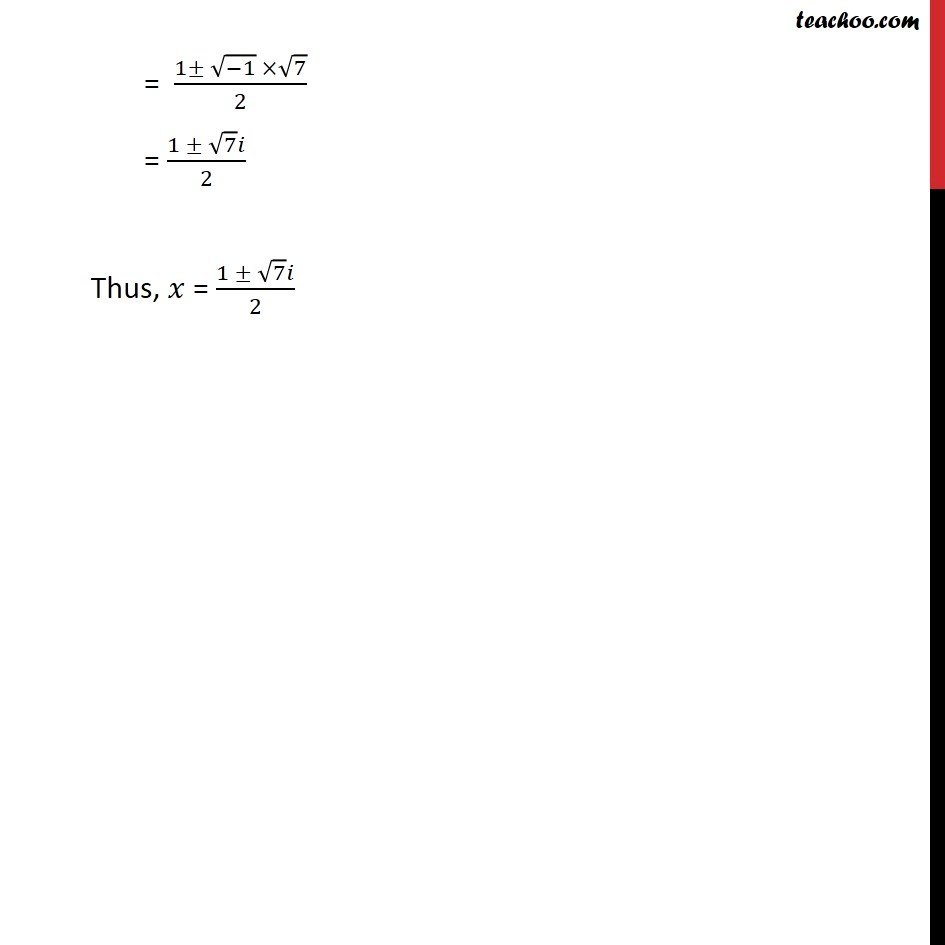Chapter 4 Class 11 Complex Numbers
Concept wiseLearn in your speed, with individual attention - Teachoo Maths 1-on-1 Class

### Transcript

Ex5.3, 6 Solve the equation 𝑥2 – 𝑥 + 2 = 0 x2 – x + 2 = 0 The above equation is of the form 𝑎𝑥2 + 𝑏𝑥 + 𝑐 = 0 Where a = 1 , b = –1 , c = 2 x = (−𝑏 ± √( 𝑏^2 −4𝑎𝑐 ))/2𝑎 x = (−(−1) ± √((−1)^2 − 4 × 1 × 2))/(2 × 1) = (1 ± √(1−8))/2 = (1 ± √(−7))/2 = (1 ± √(−1 × 7))/2 = (1± √(−1) ×√7)/2 = (1 ± √7 𝑖)/2 Thus, 𝑥 = (1 ± √7 𝑖)/2